## PLL Design

` `
`Design of a well performing Phase Locked Loop (PLL) system is not for the `
`faint hearted!. In this article I am setting down the results of my reading `
`on the subject.`
`Any engineers out there who find errors feel free to correct me. Bear in `
`mind that I have not yet got a loop to work as I would like.`
`In a single loop system there are tradeoffs between step resolution, `
`lockup time and sideband noise  A problem area is stability of the loop `
`which is not well covered In most articles on the subject. For example a `
`Motorola app note suggests you "check loop stabilty using Bode plots" but `
`does not give any further details.`
`I have built a synthesizer using a setup in another manufacturers' app note `
`and found it to be unstable, especially when modulated. `
`As I am not professionally trained I find the theory of analysis of PLLs `
`rather daunting. `
`However there are a number of programs around which will analyse the PLLs `
`for stability and other parameters. In particular, the software written by `
`KD9JQ is available freeware and gives useful info on a loop setup. `
` `
`A Phase locked loop can be represented as(Fig 1)`
` `
```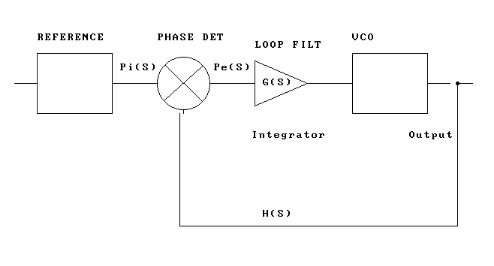Fig 1````
` `
`Pi(s) represents the phase of the reference oscillator, while Pe(S) is the`
` error phase signal which is filtered and used to drive a VCO. The transfer`
` function of this section is represented by G(S). `
`H(S) represents the feedback transfer function to the phase detector. An `
`equivalent more practical system is shown in Fig 2. `
`A Voltage Controlled Oscillator (VCO) can be swept over th frequency range `
`of interest by a control voltage. The output of the VCO is the output of `
`the PLL system which is used as the Local Oscillator of a receiver for `
`example. Some of the VCO output is fed back and compared with a reference `
`frequency in a Phase Detector (PD) The reference is usually a crystal `
`oscillator but might be the output of another loop for example. The PD `
`generates an error volatge which steers the VCO to lock it to the same `
`frequency as the reference. This simple system  produces an output on the `
`same frequency as the reference crystal oscillator. In a practical system `
`it is necessary to add a programmable divider, a reference divider and a `
```loop filter

```
` `
`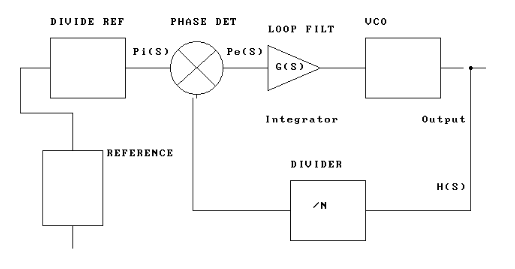Fig2`
` `
`In a typical practical system, the VCO operates at a frequency in the VHF `
`or UHF region while the reference is typically in the HF region (say 4 `
`MHz).A typical frequency step might be 25 KHz (eg in the 2M FM segment). In `
`this case the PLL reference divider will divide the crystal reference by `
`160 (4MHz -> 25 KHz). For a VCO output on (for example) 146.5 MHz the main `
`divider would be set at 5860 to divide 146.5 MHz down to 25 KHz. Thus when `
`the loop is locked, the reference and VCO signals presented to the phase `
`detector are both 25 KHz. The final component in the system is the loop `
`filter. This is necessary because a typical phase detector does not `
`generate a  "DC" error voltage but rather a pulsed waveform depending on `
`the loop lock situation. For example the Motorola MC145170 PD output is a `
`logic level signal with positive or negative going pulses. (depending on `
`how the chip is programmed).  If this waveform were applied directly to the `
`VCO a broad, frequency modulated signal would result. The loop filter `
`integrates (or averages)the PD output to produce  a smooth error voltage.`
` `
`VCO design`
`There is much debate about VCO design which is considered somewhat of a `
`"black art"! However a few principles can be stated.`
`The oscillator should use good quality components in a circuit which will `
`produce a reasonably stable low noise signal even before the PLL is `
`connected. I have found the grounded gate FET circuit works but no doubt `
`there are other opinions on the optimum circuit. The circuit inductor `
`should be as high a Q as possible. Some designs have even used a section of `
`solid coax as a transmission line inductor. In 52 MHz and 144 MHz designs I `
`have elected to use an  S18 series inductor from Cirkit in the UK.(Fig 3)`
` `
`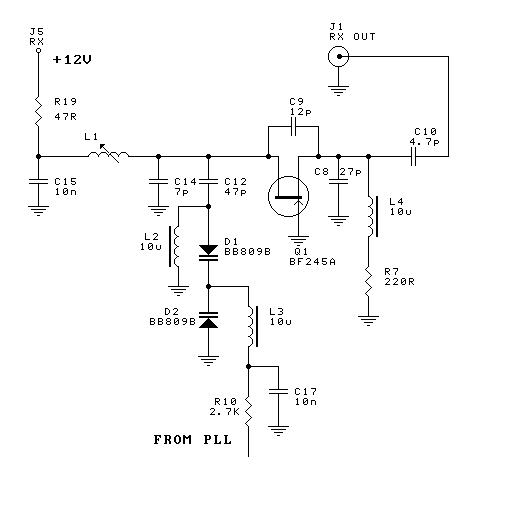`
` `
`In this circuit the values were for a 52 MHz oscillator.`
`Most VCOs are tuned with a voltage variable capacitance diode (varicap). `
`The diode is reverse biased with the VCO control voltage. These are `
`relatively low Q devices and will tend to degrade oscillator noise `
`performance. Thus they should have minimum coupling to the tuned circuit - `
`just enough to achieve an adequate frequency swing. Ideally the full tuning `
`voltage range of the diode  should be utilized. (normally 0.5-30V). The `
`tuning voltage should not be zero or the diode may be forward biased during `
`part of the oscillator cycle and degrade performance.`
`The supply for the VCO should be regulated and decoupled and the oscillator `
`should probably be shielded.  A suitable buffer circuit should isolate the `
`VCO from any load.`
`The VCO gain is important in designing the loop filter. This figure is a `
`measure of the frequency swing of the VCO per unit change in the VCO `
`control voltage.  For example, a VCO which changed frequency from 144-148 `
`MHz with a control voltage swing of 0.5 to 8.5 V would have a VCO gain of `
`0.5 MHz/Volt.`
` `
`Phase Detector`
`The phase detector is normally integrated into the PLL chip along with `
`programmable reference and main divders and digital control circuitry.  `
`There are several possible phase detector circuits.`
`For example, e 74 series EX OR gate can be configured as a phase detector. `
`Most modern PLL chips use a charge pump circuit. The output of this is a `
`logic level pulsed waveform which is integrated to produce the VCO control `
`signal.  An important parameter in designing the loop filter is the Phase `
`Detector Gain which may not be easily available from the manufacturers data `
`sheet.`
`This is a measure of the change in output voltage of the Phase Detector `
`with a change in the frequency/phase difference between the VCO and `
`reference frequencies. PD gain is expressed in Volts/Hz or Volts/Radian (1 `
`Hz = 2*pi Radians)`
` `
`Loop Filter`
`As stated above, the loop filter integrates the pulsed output from the `
`phase detector to produce a smoothed "DC" VCO control voltage. The loop `
`performance can be set by varying the component values in the loop filter.  `
```Various loop filter configurations are possible. (Fig 4)

```
` `
`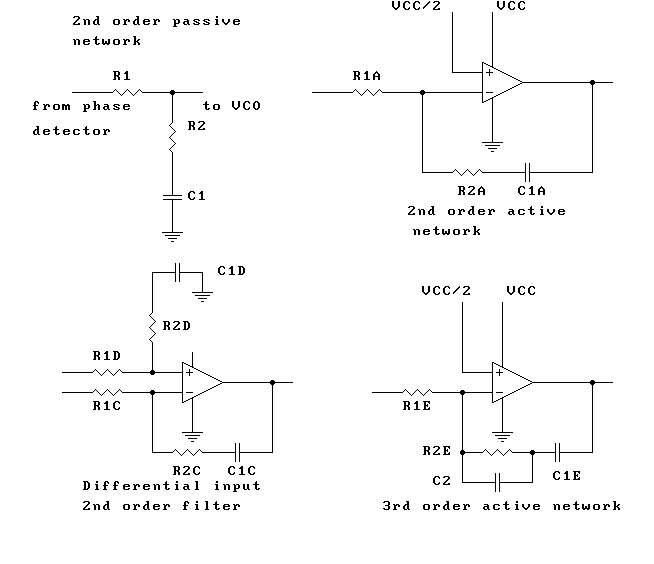`
` `
`The simplest is an RC low pass section. This will always include a second `
`resistor in series with the capacitor to "damp" the response. An `
`un/underdamped loop will "ring" after a frequency step, ie swing back and `
`forth for some time before final stable lock  is achieved. This is simplest`
`"second order" loop which is adequate for many applications. A further `
`section of RC filtering can be added to produce a third order loop with `
`improved sideband and noise performance. (C2 in the opamp circuit).`
`However it is more difficult to analyse higher order loops for stability `
`and other parameters.`
`If a simple RC filter is used, the VCO control voltage swing is limited to `
`0-5V in systems with a digital phase detector. For best phase noise `
`performance it is necessary to use the full available control range of the `
`varicap diode. To do this, a second opamp can be configured as a DC `
`amplifier to bring the output voltage to a higher figure (say 30V) (Fig 4). `
`All components in the loop filter should be designed for low noise (eg low `
`noise opamp and metal film resistors). In the example above of the 144-148 `
`MHz VCO, 1mV of noise will produce a 500 Hz swing of the VCO ouput fequency.`
` `
` `
`Programmable divider`
`The dividers are incorporated in the PLL chip in modern designs. The `
`MC145170 has a 16 bit main divider with a "legal" divide range of 40 to `
`65535. The input to the divider is buffered to accept AC coupled voltages `
`from the VCO/buffer up to 160 MHz. Earlier designs (eg MC145152) used an`
`external dual modulus prescaler to divide the VCO output down to less than `
`20 MHz. A dual modulus prescaler has two divide ratios under the control of `
`the main PLL  (eg divide by 10/11).`
`By switching between the two ratios partway through the divide cycle, the `
`PLL has much finer frequency step resolution. This is best understood by `
`looking at the maths. `
` `
`PLL control`
`Most modern designs use a serial interface from a microcontroller to load `
`data to the synthesizer.`
`A serial interface is difficult implement using EPROMs or TTL logic. This `
`makes it difficult fot hobbyists without access to a micro and suitable `
`programming skills to use these chips. `
`See my Programmable PLL board for VHF for a user `
`programmable system without the need for writing code!`
`In the case of the MC145170, a 3 wire interface is used (DATA, CLOCK and `
`ENABLE). A 24 bit serial word will update both main and reference `
`registers. An 8 bit word will update the control register which sets such `
`things as phase detector polarity, lock detect output and clock setup.`
` `
` `
`Phase noise`
`A critical parameter in PLL design is phase noise performance. Phase noise `
`is in effect frequency modulation of the VCO output by noise.`
` `
```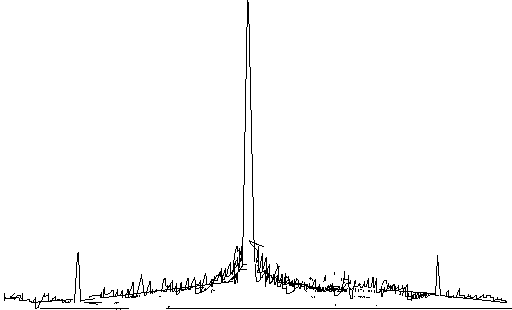```
`                               Fig 5`
` `
`Poor phase noise performance will degrade the overall system noise `
`performance in a receiver for example. Noise sidebands may mix with strong `
`adjacent signals to reduce receiver dynamic range. In a transmitter phase `
`noise will show as noise adjacent to the carrier which may interfere `
`with other services. The PLL will reduce noise outside the loop filter `
`bandwidth. Thus a low filter cutoff frequency improves phase sidebands at `
`the cost of slower lockup time. Inside the loop filter bandwidth noise is `
`determined by the VCO noise and noise added by the loop. VCO noise can be `
`minimized by a low noise transistor, run at optimum current  and high Q `
`components in the tuned circuit. PLL noise can be minmized by low noise `
`components in the loop filter and good quality supply voltage. The `
`reference to the loop will also contribute noise but in practice this is`
`not a problem where the reference is a crystal oscillator, as a crystal has `
`very good phase noise performance. `
` `
`Other spurious outputs`
`Any spurii on the reference will be reproduced in the output of the PLL. `
`This may be problem where the reference is the output of another loop or a `
`Direct Digital Synthesizer (DDS), for example. The amplitude of these spurs `
`will increased by a factor proportional to thb frequency multiplication `
`involved. The other main spurious output is related to the reference `
`itself. In the case of the 25 KHz reference example (above)  sidebands will `
`appear 25 KHz above and below the main carrier. They will be attenuated by `
`the loop filter, depending on its bandwidth.`
`Ideally the reference frequency should be at least 50 times the filter `
`cutoff.`
`   `
`Some terms`
`Various terms such as "second order"," third order" and "phase margin" are `
`used  when describing PLL design. The "order" of the loop relates to the `
`mathematical equations used to desribe the transfer function of the loop. `
`A second order loop has only one RC section in the loop `
`filter/integrator. In practice, a true second order loop does not exist as `
`there will always be additional RC sections introduced by the loop `
`amplifier open loop pole and circuit stray capacitance etc. A third `
`order loop has an additional RC pole which improves sideband `
`attenuation but makes analysis of the loop more complex. Some references `
`use a second order loop with an additional pole at approx 10 times the 2nd `
`order loop cutoff frequency. They assume the additional pole will not `
`significantly alter the phase/stability response and use a second order `
`calculation as an approximation to loop behaviour. Damping factor `
`(which describes the behaviour of the loop to a transient or step in `
`frequency) can be calculated for a second order loop but not `
`for a third. Phase margin is used to desribe loop stability in a `
`third order loop. (fig 6)`
`  `
`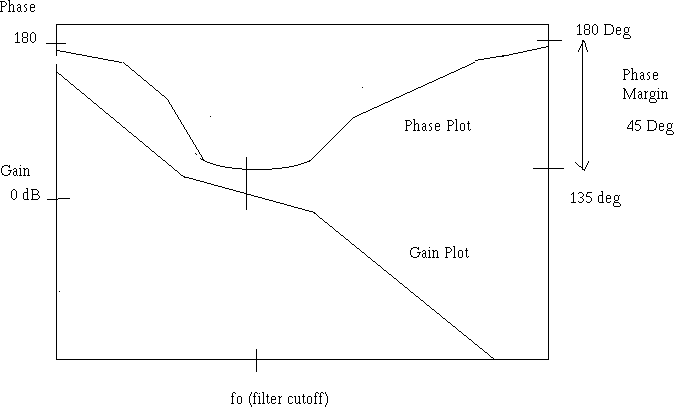`
` `
`               Fig 6`
`(Forgive the crude drawing!) Figure 6 shows the open loop gain/phase `
`response of the loop. If at filter cutoff fo loop gain is 1 ( 0 dB) or `
`greater and phase is too close to 0 (or 180 in the case of a PLL) then the `
`loop will be unstable and oscillate. The difference between 180 and the `
`actual phase response at the phase "inflection" (ie minimum) is known as `
`phase margin. Ideally it should be in the range of 30-70 degrees (45 `
`optimum). Higher phase margin will result in a more stable loop but less `
`sideband suppression.   `
` `
`Loop Design Sequence`
`Assume a synthesizer is to be designed for a 6 metre FM rig with a frequency`
`range of 50-54 MHz.  Step size will be 25 KHz over the whole band. The `
`Motorola MC145170 will cover this frequency range with minimal external `
`components.`
`Available VCO supply voltage is 12V to give a VCO control range of say 1 to `
`11V.  VCO gain Kv is thus`
` `
`(54-50)/10 =  0.4 MHz/volt or 400000 Hz/volt. `
` `
`Assume that the loop bandwidth should be a maximum of 1/50 of the reference `
`frequency to allow acceptable sidebend suppression. Maximum loop bandwidth `
`is therefore`
` `
` `
`(25000/50) = 500Hz`
` `
`Note that lockup time is inversely proportional to loop bandwidth - ie `
`higher loop bandwidth gives a quicker lockup time. Note that a higher loop `
`bandwidth will mean less reference sideband suppression and but better `
`close in VCO phase noise suppression. For an amateur receiver, loop lockup `
`time is not critical and anything less than 10-20 msec would be acceptable. `
`We will thus aim for a loop Fo (loop filter cutoff - approx equal to 3 dB `
`bandwidth) of about 100Hz.`
` `
`Kp or phase detector gain is VDD/4pi for the single ended output and VDD/2Pi `
`for the differential output of the MC145170. `
`For a 5V supply (VDD) Kp is 0.398 or approx 0.4 for the single ended and 0.8 `
`for the differential output. I have elected to use a 2nd order filter circuit `
`with an additional opamp for DC gain. This allows a wider VCO control `
`voltage range and eases the design of the loop  `
`The circuit of the PLL is shown in `
` `
```Fig 7.

```
` `
`I have cheated on the loop calculations and used the freeware program from `
```KD9JQ

to calculate the ```
`loop components for a 2nd order PLL with the following parameters:`
` `
`Freq range                                                         50-54 MHz`
`Freq step                                                            25000 Hz`
`Loop Type                                                          1 (active)`
`Bandwidth                                                          100 Hz`
`Damping factor                                                  0.7`
`VCO Gain (Kv)                                                  400000 Hz/V`
`PD gain(Kp)                                                       0.8 (differential output)                       `
`Phase det type                                                  6 (tristate FF)`
`DC Gain (Ka)                                                     2.6`
`Division (N)                                                        2080 (midband figure)`
`Opamp open loop gain                                     100000`
`Ref freq                                               25000 Hz`
` `
`The initial value of R2(damping resistor) was entered as 2200 Ohms`
`and the other values adjusted to 12 K and 2.2 uF as in the circuit`
`With these values and the above figures, the following results`
`were obtained:`
` `
`Bandwidth                                                          103 Hz`
`Locktime                                                            3.2 msec`
`Reference  suppression                                   -50.7 dB`
`VCO noise suppression                                   -27.7 dB`
`Damping factor                                                  0.75`
` `
` `
`I have built this circuit for a 6 meter transceiver project. Using a `
`spectrum analyser to observe loop behaviour it appears to be stable `
`and behave predictably. I have not formally measured phase noise performance`
`but it looks OK on the spectrum analyser!`